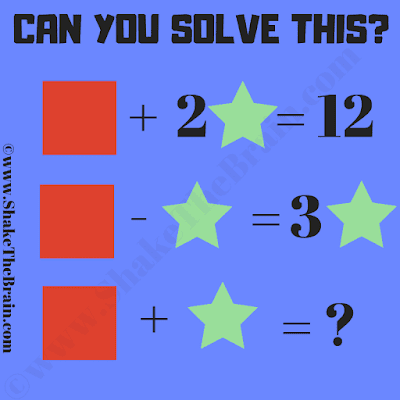This is an easy maths equation brainteaser for school students. Solving these simultaneous equations will help you to improve your maths skills. In this math brain teaser find the value of the Square and Star and then solve the last equation.Can you solve this?
The answer to this "Simple Math Equation Brain Teaser for Students", can be viewed by clicking on the answer button.